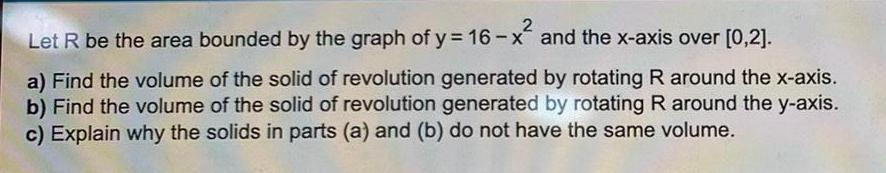Question:

# Let R be the area bounded by the graph of y = 16-x² and the

Last updated: 8/14/2022Let R be the area bounded by the graph of y = 16-x² and the x-axis over [0,2]. a) Find the volume of the solid of revolution generated by rotating R around the x-axis. b) Find the volume of the solid of revolution generated by rotating R around the y-axis. c) Explain why the solids in parts (a) and (b) do not have the same volume.